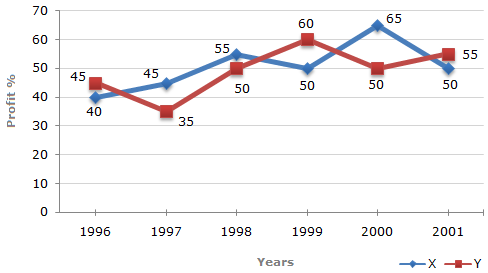# Data Interpretation - Line Charts - Discussion

Discussion Forum : Line Charts - Line Chart 4 (Q.No. 5)
Directions to Solve

The following line graph gives the percent profit earned by two Companies X and Y during the period 1996 - 2001.

Percentage profit earned by Two Companies X and Y over the Given Years

 %Profit = Income - Expenditure x 100 Expenditure5.
If the incomes of two Comapanies were equal in 1999, then what was the ratio of expenditure of Company X to that of Company Y in 1999 ?
6:5
5:6
11:6
16:15
Explanation:

Let the incomes of each of the two Companies X and Y in 1999 be Rs. x.

And let the expenditures of Companies X and Y in 1999 be E1 and E2 respectively.

Then, for Company X we have:

 50 = x - E1 x 10050 = x - 1x = 150 E1 .... (i) E1 100 E1 100

Also, for Company Y we have:

 60 = x - E2 x 10060 = x - 1x = 160 E2 .... (ii) E2 100 E2 100

From (i) and (ii), we get:

 150 E1 = 160 E2E1 = 160 = 16 (Required ratio). 100 100 E2 150 15

Discussion:
3 comments Page 1 of 1.

Rahul agarwal said:   1 decade ago
More easiest way to solve this question will be
Let Income of both X and Y be 240
240/15:240/16=16:15

Bhavin said:   9 years ago
@Rahul. Why have you assumed income as 240? and why not any other number?

Gaurav Gavankar said:   6 years ago
From profit formula, we can write expenditure=(income*100)/(profit+100) . Now calculate ratio of expenditures of both companies.

i.e. E(X)/E(Y)= {(income(x)*100)/(profit(x)+100) }/ { (income(y)*100)/(profit(y)+100)}.

As, income(x) = income(y).

Therefore E(X)/E(Y) = (profit(y) + 100)/(profit(x) + 100).
= (60+100)/(50+100).
= 160/150.
E(X)/E(Y) = 16:15.